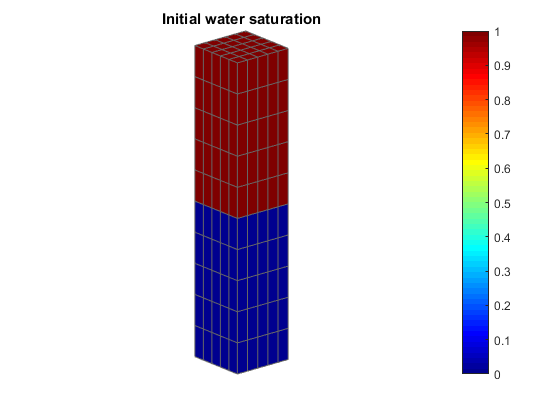#Gravity segregation

## Gravity segregation using two phase AD solvers

This example demonstrates a simple, but classical test problem for a multiphase solver. The problem we are considering is that of gravity segregation: For a given domain we let the initial fluid distribution consist of a dense fluid atop of a lighter one. Gravity is the dominating force and over time, the model goes towards the stable equilibrium where the denser fluid has switched place with the lighter fluid.

The example also demonstrates how to set up a simple schedule (one or more timesteps with a collection of driving forces such as bc and wells).

We begin by defining a simple homogenous reservoir of 1 by 1 by 5 meters and 250 simulation cells.

```mrstModule add ad-core ad-blackoil ad-props mrst-gui

dims = [5 5 10];
G = cartGrid(dims, [1, 1, 5]*meter);
G = computeGeometry(G);

rock = makeRock(G, 100*milli*darcy, 0.5);
```

## Defining the fluid properties

In this section we set up a generic fluid suitable for solvers based on automatic differentiation. The fluid model is by default incompressible. We define all three phases (Water, oil and gas), but we will only use the first two in practice.

```fluid = initSimpleADIFluid('mu', [1, 10, 1]*centi*poise, ...
'n',  [1 1 1], ...
'rho', [1000, 700, 250]*kilogram/meter^3);
```

## Defining the model

The model contains all the necessary functions to simulate a mathematical model using the AD-solvers. In our case, we are interested in a standard fully implicit two phase oil/water model. By passing the grid, rock and fluid into the model constructor we obtain a model with precomputed operators and quantities that is ready for simulation. We also ensure that gravity is enabled before we call the constructor.

```gravity reset on
model = TwoPhaseOilWaterModel(G, rock, fluid);

% Show the model
disp(model)
```
```  TwoPhaseOilWaterModel with properties:

disgas: 0
vapoil: 0
drsMaxRel: Inf
drsMaxAbs: Inf
fluid: [1x1 struct]
rock: [1x1 struct]
dpMaxRel: Inf
dpMaxAbs: Inf
...```

## Set up initial state

We must also define the initial state. The state contains the unstable initial fluid distribution. We do this by setting the water saturation to 1 in all cells and then setting it to zero in cells below a threshold.

We defined the water (first phase) density to be 1000 kg/m^3 when we set up the fluid model. Note that we let the pressure be uniform initially. The model is relatively simple, so we do not need to worry abot the initial pressure being reasonable, but it is something that should be considered for models with for example compressibility.

To ensure that we got the distribution right, we make a plot of the initial saturation.

```[ii, jj, kk] = gridLogicalIndices(G);

lower = kk > 5;
sW = ones(G.cells.num, 1);
sW(lower) = 0;
s = [sW, 1 - sW];
% Finally set up the state
state = initResSol(G, 100*barsa, s);

% Plot the initial water saturation
figure;
plotCellData(G, state.s(:, 1))
axis equal tight off
view(50, 20)

title('Initial water saturation')
colorbar
```## Set up boundary conditions and timesteps

Finally, we add a simple boundary condition of 100 bar pressure at the bottom of the reservoir. This is not strictly required, but the incompressible model will produce singular linear systems if we do not fix the pressure somehow. It is well known that the pressure is not unique for an incompressible model if there are no Dirichlet boundary conditions, as multiple pressures will give the same velocity field.

Another alternative would be to add (some) compressibility to our fluid model.

Once we have set up the boundary conditions, we define 20 timesteps of 90 days each which is enough for the state to approach equilibrium. The simulator will cut timesteps if they do not converge, so we are not overly worried about the large initial timestep when the problem is the most stiff.

Finally, we combine the timesteps and the boundary conditions into a schedule. A schedule collects multiple timesteps into a single struct and makes it easier to have for example different boundary conditions at different time periods. For this example, however, the schedule is very simple.

```bc = [];
bc = pside(bc, G, 'ZMin', 100*barsa, 'sat', [0 1]);

dt = repmat(90*day, 20, 1);

schedule = simpleSchedule(dt, 'bc', bc);
```

## Simulate the problem

Finally, we simulate the problem. We note that the three simple objects define the entire simulation:

• state defines the initial values in the reservoir
• model defines the partial differential equations, their physical constraints and the numerical discretizations required to advance from one time to the next.
• schedule defines any time dependent input parameters as well as the timesteps.
```[wellSols, states] = simulateScheduleAD(state, model, schedule, 'Verbose', true);
```

Published May 1, 2015